# Important Questions Class 9 Maths Chapter 10 - Circles

Important Questions Class 9 Maths Chapter 10 Circles are provided as per NCERT book and CBSE syllabus. These questions have been provided by our subject experts to help students score good marks in their final exam. Also, there are some extra questions given in the below section for better practice and revision.

The chapter circles will include concepts of circumscribed and inscribed and questions based on them. Practice all chapters of class 9 Maths important questions to score excellent marks in this subject. These CBSE Class 9 Maths Chapter 10 Circles important questions set the basis for the final question paper and help students to face exams with more confidence.

Also Check:

## Important Questions Class 9 Maths Chapter 10 Circles with Solutions

Question 1:

Bisectors of angles A, B and C of a triangle ABC intersect its circumcircle at D, E and F respectively. Prove that the angles of the triangle DEF are 90° – (½)A, 90° – (½)B and 90° – (½)C.

Solution:

Consider the following diagram: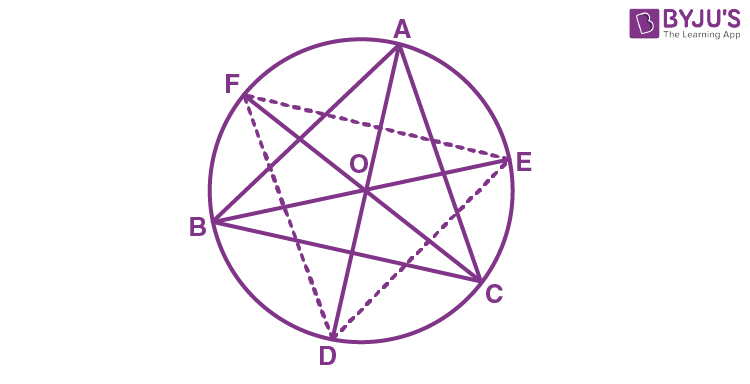Here, ABC is inscribed in a circle with center O and the bisectors of ∠A, ∠B and ∠C intersect the circumcircle at D, E and F respectively.

Now, join DE, EF and FD

As angles in the same segment are equal, so,

∠FDA = ∠FCA ————-(i)

∠FDA = ∠EBA ————-(i)

Adding equations (i) and (ii) we have,

∠FDA + ∠EDA = ∠FCA + ∠EBA

Or, ∠FDE = ∠FCA + ∠EBA = (½)∠C + (½)∠B

We know, ∠A + ∠B + ∠C = 180°

So, ∠FDE = (½)[∠C + ∠B] = (½)[180° – ∠A]

⇒ ∠FDE = [90 – (∠A/2)]

In a similar way,

∠FED = [90 – (∠B/2)]

And,

∠EFD = [90 – (∠C/2)]

Question 2:

In any triangle ABC, if the angle bisector of ∠A and perpendicular bisector of BC intersect, prove that they intersect on the circumcircle of the triangle ABC.

Solution:

Consider this diagram: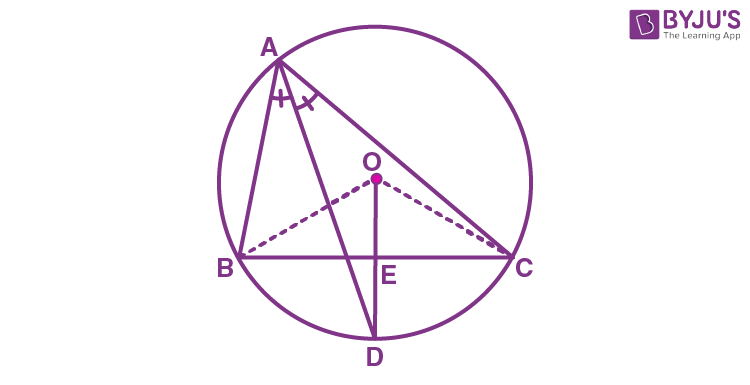Given: In ∆ABC, AD is the angle bisector of ∠A and OD is the perpendicular bisector of BC, intersecting each other at point D.
To Prove: D lies on the circle
Construction: Join OB and OC
Proof:
BC is a chord of the circle.
The perpendicular bisector will pass through centre O of the circumcircle.
∴ OE ⊥ BC & E is the midpoint of BC
Chord BC subtends twice the angle at the centre, as compared to any other point.
BC subtends ∠BAC on the circle & BC subtends ∠BOC on the centre
∴ ∠BAC = 1/2 ∠ BOC
In ∆ BOE and ∆COE,
BE = CE (OD bisects BC)
∠BEO = ∠CEO (Both 90°, as OD ⊥ BC)
OE = OE (Common)
∴ ∆BOE ≅ ∆COE (SAS Congruence rule)
∴ ∠BOE = ∠COE (CPCT)
Now,
∠BOC = ∠BOE + ∠COE
∠BOC = ∠BOE + ∠BOE
∠BOC = 2 ∠BOE …(2)
AD is angle bisector of ∠A
From (1)
∠BAC = 1/2 ∠BOC
BD subtends ∠BOE at centre and half of its angle at Point A.
Hence, BD must be a chord.
∴ D lies on the circle.

Question 3:

Prove that the circle drawn with any side of a rhombus as diameter passes through the point of intersection of its diagonals.

Solution: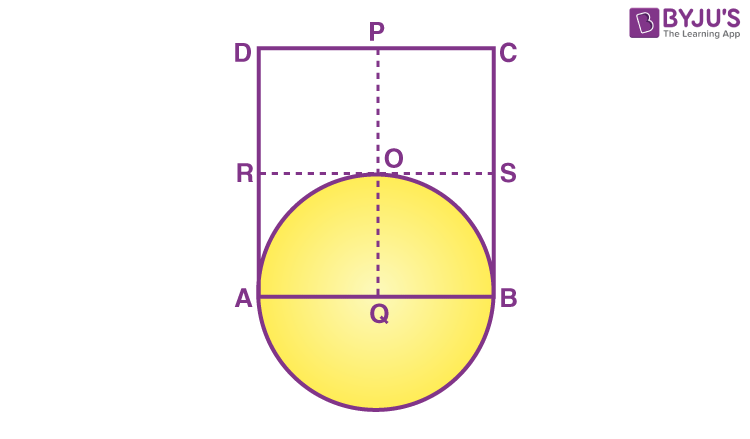To prove: A circle drawn with Q as centre, will pass through A, B and O (i.e. QA = QB = QO)

Since all sides of a rhombus are equal,

AB = DC

Now, multiply (½) on both sides

(½)AB = (½)DC

So, AQ = DP

⇒ BQ = DP

Since Q is the midpoint of AB,

AQ= BQ

Similarly,

RA = SB

Again, as PQ is drawn parallel to AD,

RA = QO

Now, as AQ = BQ and RA = QO we have,

QA = QB = QO (hence proved).

Question 4:

Two chords AB and CD of lengths 5 cm and 11 cm respectively of a circle are parallel to each other and are on opposite sides of its centre. If the distance between AB and CD is 6, find the radius of the circle.

Solution: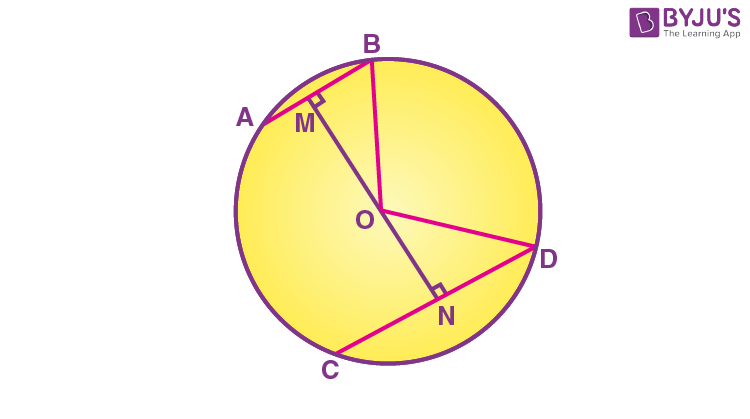Here, OM ⊥ AB and ON ⊥ CD. is drawn and OB and OD are joined.

As we know, AB bisects BM as the perpendicular from the centre bisects the chord.

Since AB = 5 so,

BM = AB/2

Similarly, ND = CD/2 = 11/2

Now, let ON be x.

So, OM = 6− x.

Consider ΔMOB,

OB2 = OM2 + MB2

Or,

OB2 = 36 + x2 – 12x + 25/4 ……(1)

Consider ΔNOD,

OD2 = ON2 + ND2

Or,

OD2 = x2+121/4 ……….(2)

We know, OB = OD (radii)

From eq. (1) and eq. (2) we have;

36 + x2 -12x + 25/4 = x2 + 121/4

12x = 36 + 25/4 – 121/4

12x = (144 + 25 -121)/4

12x = 48/4 = 12

x = 1

Now, from eq. (2) we have,

OD2 = 11 + (121/4)

Or OD = (5/2) × √5

Question 5:

If circles are drawn taking two sides of a triangle as diameters, prove that the point of intersection of these circles lies on the third side.

Solution:

First, draw a triangle ABC and then two circles having a diameter as AB and AC respectively.

We will have to now prove that D lies on BC and BDC is a straight line.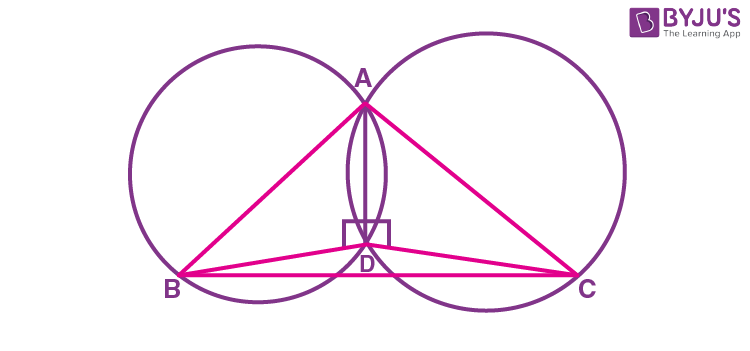Proof:

As we know, angle in the semi-circle are equal

∴ ∠BDC is a straight line.

So, it can be said that D lies on the line BC.

Question 6:

If the non-parallel sides of a trapezium are equal, prove that it is cyclic.

Solution:

Construction-Consider a trapezium ABCD with AB||CD and BC = AD.

Draw AM ⊥CD and BN ⊥ CD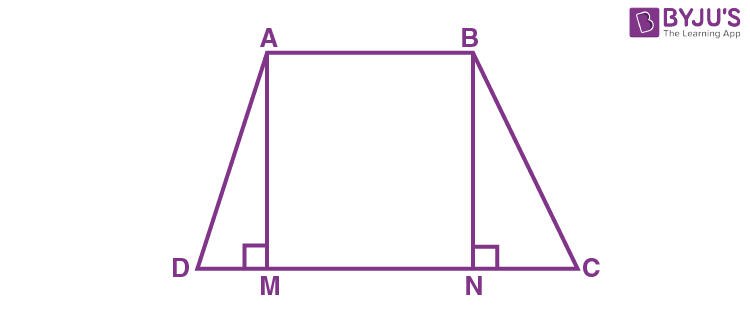In ∆AMD and ∆BNC;

∠AMD = ∠BNC (90°)

AM =BN (perpendiculars between parallel lines)

∆AMD = ∆BNC (By RHS congruency)

∆ADC = ∆BCD (By CPCT rule) …….(i)

∠BAD + ∠BCD = 180° (by equation (i))

Since, the opposite angles are supplementary, therefore, ABCD is a cyclic quadrilateral.

Question 7:

ABCD is a cyclic quadrilateral whose diagonals intersect at a point E. If ∠DBC = 70°, ∠BAC is 30°, find ∠BCD. Further, if AB = BC, find ∠ECD.

Solution:

Consider the following diagram.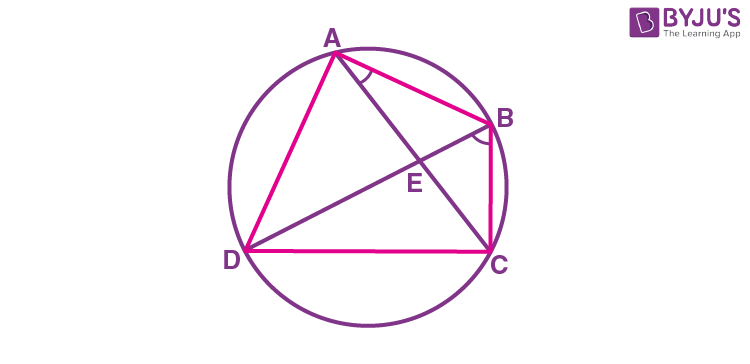Consider the chord CD,

As we know, angles in the same segment are equal.

Now, ∠BAD will be equal to the sum of angles BAC and CAD.

= 30° + 70°

As we know, the opposite angles of a cyclic quadrilateral sum up to 180 degrees.

So,

So, ∠BCD = 80°

Now consider the ΔABC.

Here, it is given that AB = BC

Also, ∠BCA = ∠CAB (Angles opposite to equal sides of a triangle)

∠BCA = 30°

also, ∠BCD = 80°

∠BCA + ∠ACD = 80°

So, ∠ACD = 50° and,

∠ECD = 50°

Question 8:

In Figure, ∠ABC = 69°, ∠ ACB = 31°, find ∠BDC.

Solution: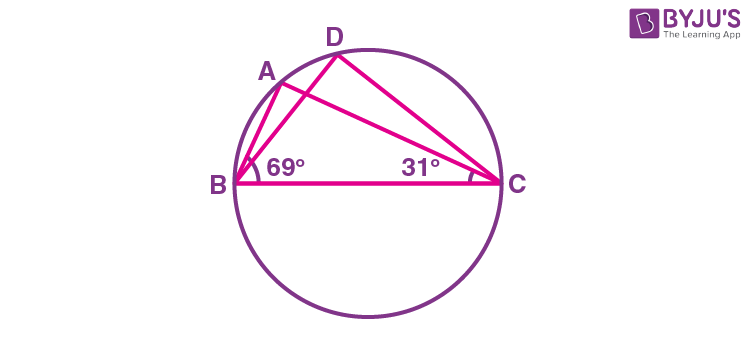As we know, angles in the segment of the circle are equal so,

∠BAC = ∠BDC

Now in the In ΔABC, sum of all the interior angles will be 180°

So, ∠ABC + ∠BAC + ∠ACB = 180°

Now, by putting the values,

∠BAC = 180° – 69° – 31°

So, ∠BAC = 80°

Question 9:

In Figure, ∠PQR = 100°, where P, Q and R are points on a circle with centre O. Find ∠OPR.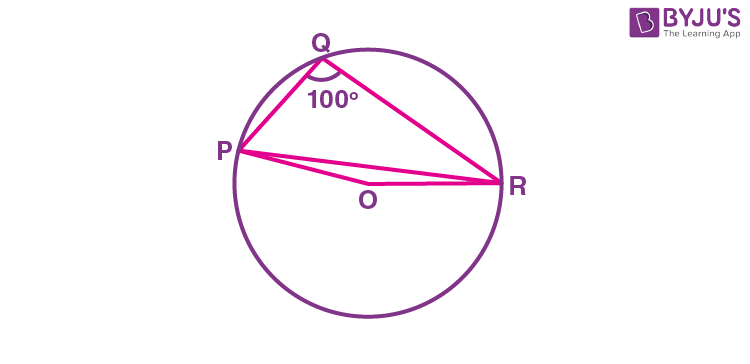Solution:

Since angle which is subtended by an arc at the centre of the circle is double the angle subtended by that arc at any point on the remaining part of the circle.

So, the reflex ∠POR = 2 × ∠PQR

We know the values of angle PQR as 100°

So, ∠POR = 2 × 100° = 200°

∴ ∠POR = 360° – 200° = 160°

Now, in ΔOPR,

OP and OR are the radii of the circle

So, OP = OR

Also, ∠OPR = ∠ORP

Now, we know sum of the angles in a triangle is equal to 180 degrees

So,

∠POR + ∠OPR + ∠ORP = 180°

⇒ ∠OPR + ∠OPR = 180° – 160°

As ∠OPR = ∠ORP

⇒ 2∠OPR = 20°

Thus, ∠OPR = 10°

Question 10:

A circular park of radius 20m is situated in a colony. Three boys Ankur, Syed and David are sitting at equal distance on its boundary each having a toy telephone in his hands to talk each other. Find the length of the string of each phone.

Solution:

First, draw a diagram according to the given statements. The diagram will look as follows.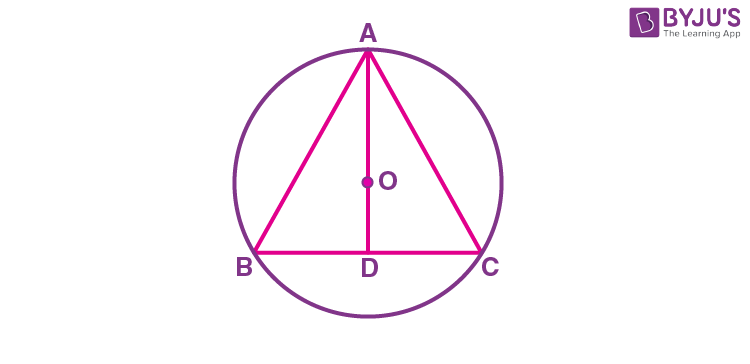Here the positions of Ankur, Syed and David are represented as A, B and C respectively. Since they are sitting at equal distances, the triangle ABC will form an equilateral triangle.

AD ⊥ BC is drawn. Now, AD is median of ΔABC and it passes through the centre O.

Also, O is the centroid of the ΔABC. OA is the radius of the triangle.

Let the side of a triangle a metres then BD = a/2 m.

Applying Pythagoras theorem in ΔABD,

⇒ AD2 = AB2 – BD2

⇒ AD2 = a2 – (a/2)2

⇒ 20 m = 2/3 × √3a/2

⇒ a = 20√3 m

So, the length of the string of the toy is 20√3 m.

Question 11:

If two equal chords of a circle intersect within the circle, prove that the line joining the point of intersection to the centre makes equal angles with the chords.

Solution:

From the question we have the following conditions:

(i) AB and CD are 2 chords which are intersecting at point E.

(ii) PQ is the diameter of the circle.

(iii) AB = CD.

Now, we will have to prove that ∠BEQ = ∠CEQ

For this, the following construction has to be done:

Construction:

Draw two perpendiculars are drawn as OM ⊥ AB and ON ⊥ CD. Now, join OE. The constructed diagram will look as follows: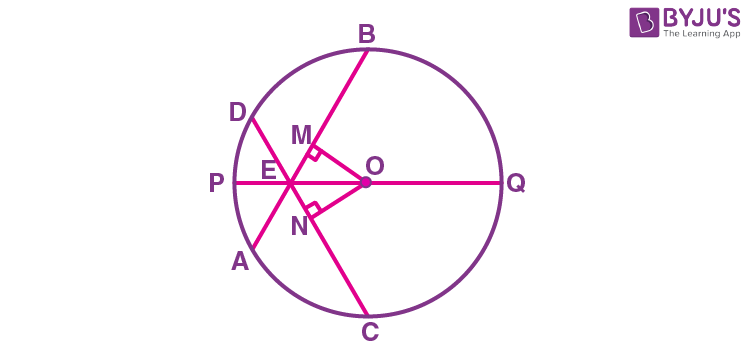Now, consider the triangles ΔOEM and ΔOEN.

Here,

(i) OM = ON [Since the equal chords are always equidistant from the centre]

(ii) OE = OE [It is the common side]

(iii) ∠OME = ∠ONE [These are the perpendiculars]

So, by RHS similarity criterion, ΔOEM ≅ ΔOEN.

Hence, by CPCT rule, ∠MEO = ∠NEO

∴ ∠BEQ = ∠CEQ (Hence proved).

Question 12:

If two circles intersect at two points, prove that their centres lie on the perpendicular bisector of the common chord.

Solution: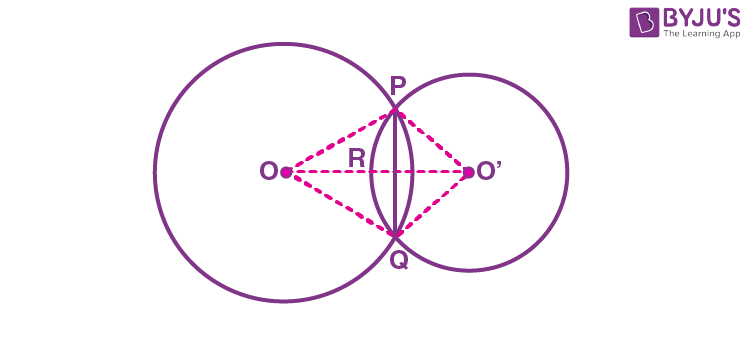It is given that two circles intersect each other at P and Q.

To prove:

OO’ is a perpendicular bisector of PQ.

Proof:

Triangle ΔPOO’ and ΔQOO’ are similar by SSS congruency since

OP = OQ and O’P = O’Q (Since they are also the radii)

OO’ = OO’ (It is the common side)

So, It can be said that ΔPOO’ ≅ ΔQOO’

∴ ∠POO’ = ∠QOO’ — (i)

Even triangles ΔPOR and ΔQOR are similar by SAS congruency as

∠POR = ∠QOR (As ∠POO’ = ∠QOO’)

OR = OR (Common arm)

So, ΔPOR ≅ ΔQOR

∴ ∠PRO = ∠QRO

Also, As we know,

∠PRO + ∠QRO = 180°

Hence, ∠PRO = ∠QRO = 180°/2 = 90°

So, OO’ is the perpendicular bisector of PQ.

Question 13:

Prove that if chords of congruent circles subtend equal angles at their centres, then the chords are equal.

Solution:

Consider the following diagram-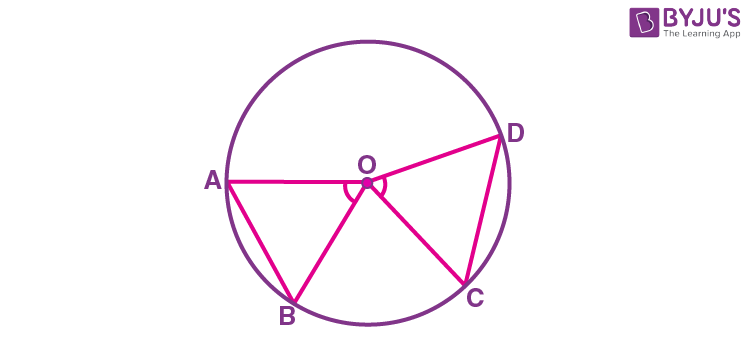Here, it is given that ∠AOB = ∠COD i.e. they are equal angles.

Now, we will have to prove that the line segments AB and CD are equal i.e. AB = CD.

Proof:

In triangles AOB and COD,

∠AOB = ∠COD (as given in the question)

OA = OC and OB = OD ((these are the radii of the circle)

So, by SAS congruency, ΔAOB ≅ ΔCOD.

∴ By the rule of CPCT, AB = CD. (Hence proved).

## Video Lessons on Circles

### Introduction to Circles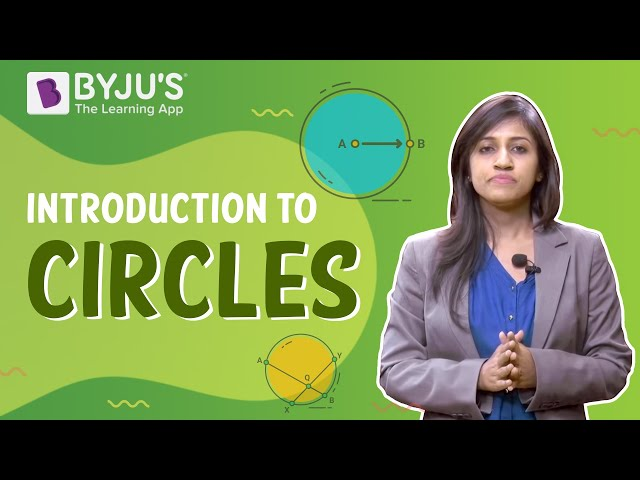### Parts of a Circle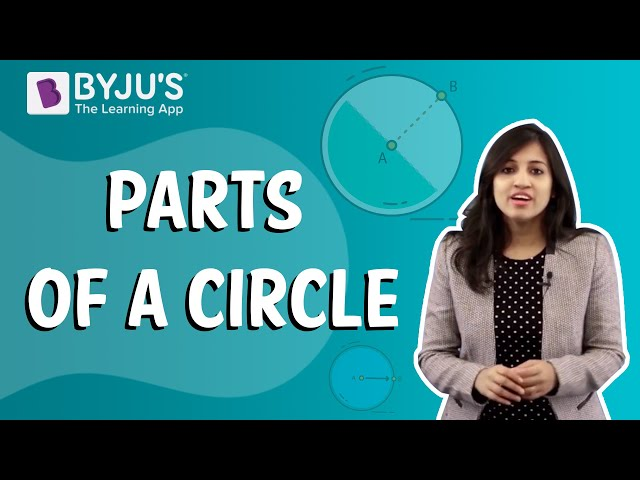### Area of a Circle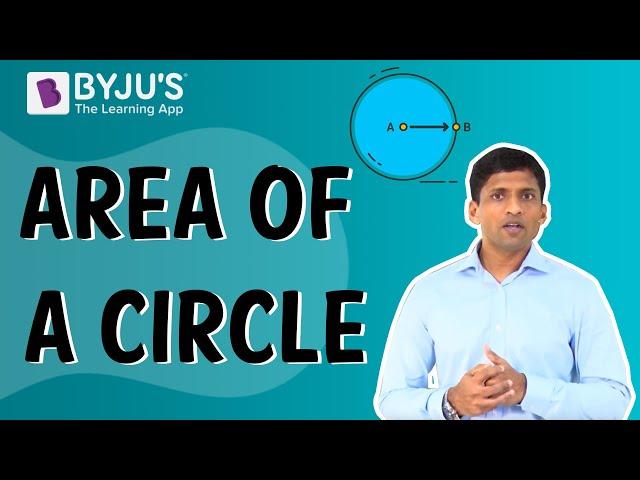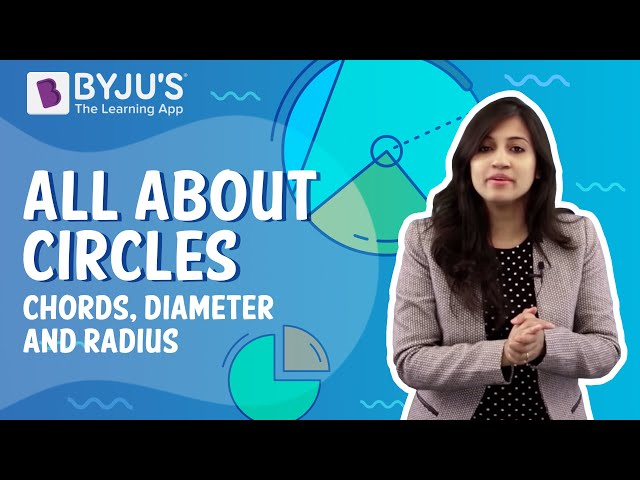### Important Questions For Class 9 Maths Chapter 10 Circles – Practice Questions

Solve the below given important questions class 9 Maths chapter 10 circles to score good marks in the final examination.

1. Recall that two circles are congruent if they have the same radii. Prove that equal chords of congruent circles subtend equal angles at their centres.
2. Two circles of radii 5 cm and 3 cm intersect at two points and the distance between their centres is 4 cm. Find the length of the common chord.
3. If two equal chords of a circle intersect within the circle, prove that the segments of one chord are equal to corresponding segments of the other chord.
4. If a line intersects two concentric circles (circles with the same centre) with centre O at A, B, C and D, prove that AB = CD
5. Three girls Reshma, Salma and Mandip are playing a game by standing on a circle of radius 5m drawn in a park. Reshma throws a ball to Salma, Salma to Mandip, Mandip to Reshma. If the distance between Reshma and Salma and between Salma and Mandip is 6m each, what is the distance between Reshma and Mandip?
6. In the given figure, A, B and C are three points on a circle with centre O such that ∠BOC = 30° and ∠AOB = 60°. If D is a point on the circle other than the arc ABC, find ∠ADC.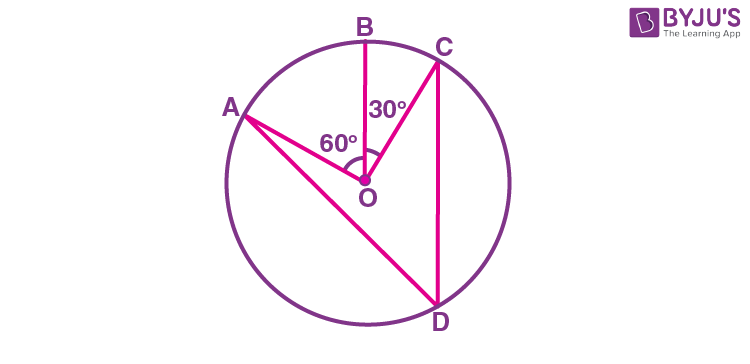7. A chord of a circle is equal to the radius of the circle. Find the angle subtended by the chord at a point on the minor arc and also at a point on the major arc.
8. Prove that a cyclic parallelogram is a rectangle.
9. Let the vertex of an angle ABC be located outside a circle and let the sides of the angle intersect equal chords AD and CE with the circle. Prove that ∠ABC is equal to half the difference of the angles subtended by the chords AC and DE at the centre.
10. ABCD is a parallelogram. The circle through A, B and C intersect CD (produced if necessary) at E. Prove that AE, = AD.
11. AC and BD are chords of a circle which bisect each other. Prove that (i) AC and BD are diameters; (ii) ABCD is a rectangle.

Keep visiting BYJU’S – The Learning app and download the app to get all the class-wise important questions and learn all Maths-related concepts quickly by exploring more videos.